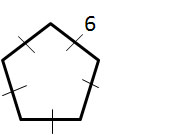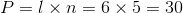# High School Math : How to find the perimeter of a pentagon

## Example Questions

### Example Question #1 : Pentagons

What is the perimeter of the pentagon?Possible Answers:Correct answer:Explanation:

The perimeter of a polygon is found by calculating the sum of all of the side lengths. In this instance, the polygon is regular, so the perimeter can be found by mulitplying the length of one side by the total number of sides:### All High School Math Resources